# imshow

## 语法

``imshow(I)``
``imshow(I,[low high])``
``imshow(I,[])``
``imshow(RGB)``
``imshow(BW)``
``imshow(X,map)``
``imshow(filename)``
``imshow(___,Name=Value)``
``himage = imshow(___)``

## 说明

``imshow(I)` 在图窗中显示灰度图像 `I`。`imshow` 使用图像数据类型的默认显示范围，并优化图窗、坐标区和图像对象属性以便显示图像。`
``imshow(I,[low high])` 显示灰度图像 `I`，以二元素向量 `[low high]` 形式指定显示范围。有关详细信息，请参阅 `DisplayRange` 参数。`

``imshow(I,[])` 显示灰度图像 `I`，根据 `I` 中的像素值范围对显示进行转换。`imshow` 使用 `[min(I(:)) max(I(:))]` 作为显示范围。`imshow` 将 `I` 中的最小值显示为黑色，将最大值显示为白色。有关详细信息，请参阅 `DisplayRange` 参数。`

``imshow(RGB)` 在图窗中显示真彩色图像 `RGB`。`

``imshow(BW)` 在图窗中显示二值图像 `BW`。对于二值图像，`imshow` 将值为 `0`（零）的像素显示为黑色，将值为 `1` 的像素显示为白色。`

``imshow(X,map)` 显示带有颜色图 `map` 的索引图像 `X`。`

``imshow(filename)` 显示存储在由 `filename` 指定的图形文件中的图像。`
``imshow(___,Name=Value)` 使用名称-值参数控制运算的各个方面来显示图像。 `
``himage = imshow(___)` 返回 `imshow` 创建的图像对象。`

## 示例

`rgbImage = imread("peppers.png");`

`imshow(rgbImage)``grayImage = im2gray(rgbImage);`

`imshow(grayImage)`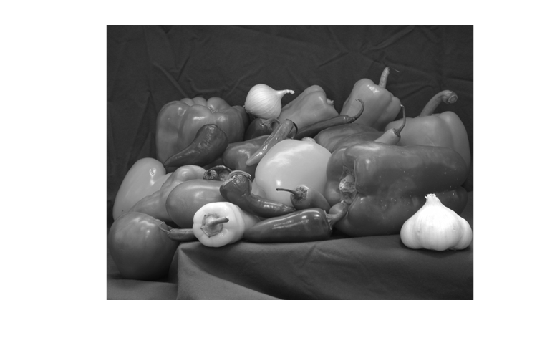```meanVal = mean(grayImage,"all"); binaryImage = grayImage >= meanVal;```

`imshow(binaryImage)`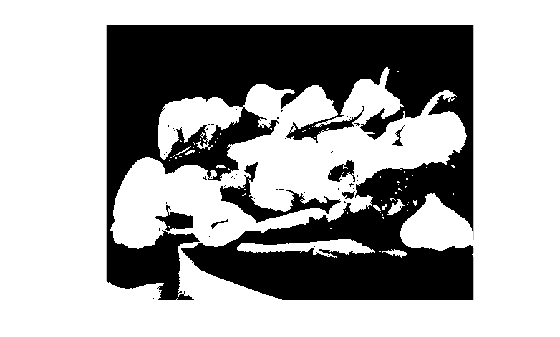`[corn_indexed,map] = imread("corn.tif");`

`imshow(corn_indexed,map)`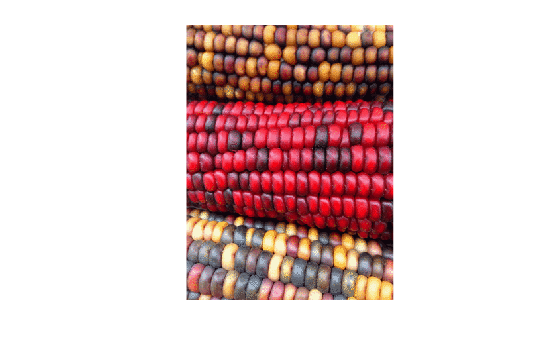```filename = "peppers.png"; imshow(filename)``````load("mri.mat"); vol = squeeze(D);```

```sliceZ = vol(:,:,13); imshow(sliceZ,[],Colormap=copper)```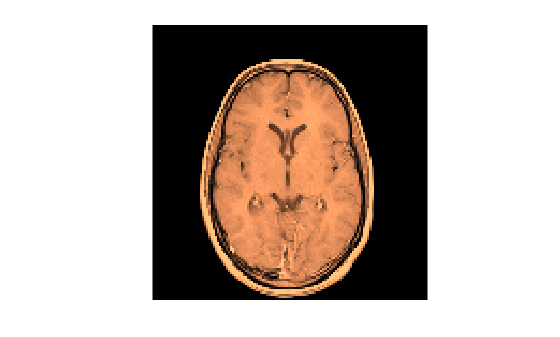`colormap(hot)`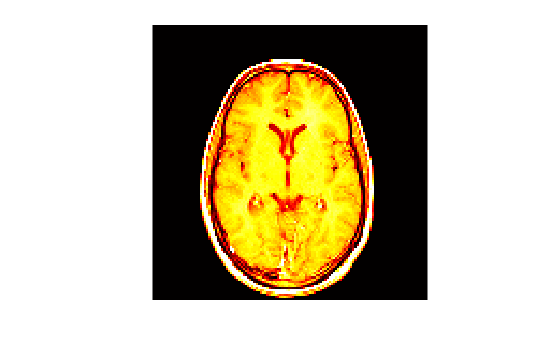`RGB = imread("peppers.png");`

```G = RGB(:,:,2); imshow(G)```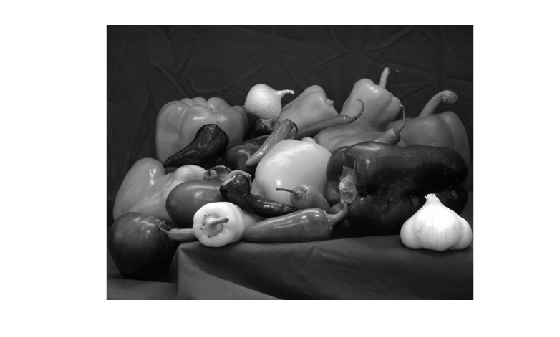`filt = [-1 -1 -1; 0 0 0; 1 1 1];`

`edgeG = filter2(filt,G);`

`imshow(edgeG)`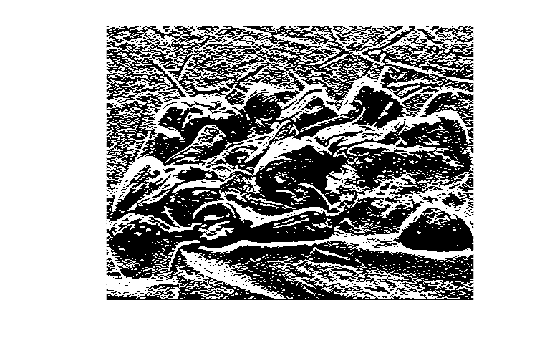`imshow(edgeG,[])`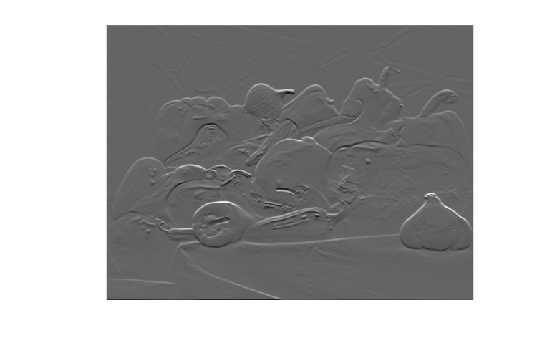`corn.tif` 文件中的灰度图像读取到工作区中。此图像的灰度版本是文件中的第二个图像。

`corn_gray = imread("corn.tif",2);`

```corn_detail = corn_gray(1:100,1:100); imshow(corn_detail)```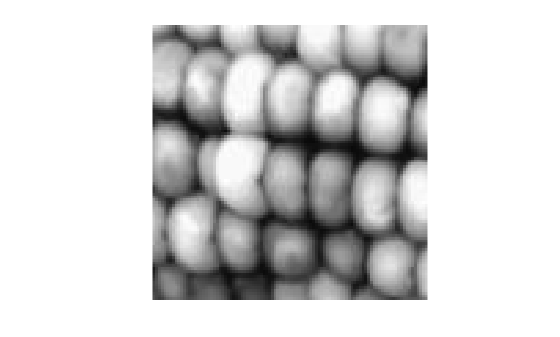`imshow(corn_detail,"InitialMagnification",1000)``imshow(corn_detail,"InitialMagnification",1000,"Interpolation","bilinear")`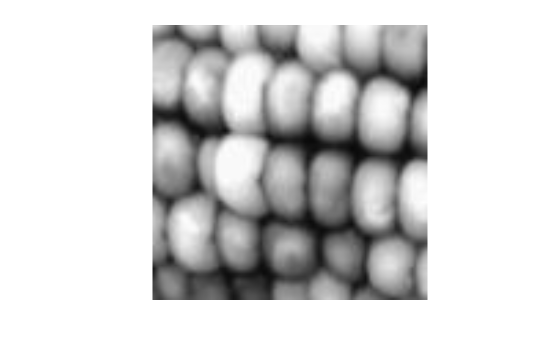## 输入参数

### 名称-值参数

• 如果 `I` 是整数类型，则 `DisplayRange` 默认为该整数类的最小和最大可表示值。例如，`uint16` 数组的默认显示范围是 [0, 65535]。

• 如果 `I` 的数据类型为 `single``double`，则默认显示范围是 [0, 1]。

MATLAB Online™ 中，`InitialMagnification` 设置为 `"fit"` 且不能更改。

• `"nearest"` - 最近邻点插值。位于 (x, y) 的像素值是原始图像中与 (x, y) 最邻近的像素值。当有少量表示明显不同类别的像素值时，或当您要在高度放大的视图中查看各个像素时，此方法最合适。

• `"bilinear"` - 双线性插值。位于 (x, y) 的像素值是原始图像中该位置周围像素的加权平均值。为了最大限度地减少显示伪影，当您缩小图像时，`imshow` 函数会执行抗锯齿功能。此方法在几乎所有其他情况下都最合适。

## 输出参数

`imshow` 函数创建的图像，指定为图像对象。

## 提示

• 要在创建图像后更改颜色图，请使用 `colormap` 命令。

• 可以将 `imshow``tiledlayout``nexttile` 函数结合使用，在同一个图窗中显示多个具有不同颜色图的图像。

• 您可以在调用 `imshow` 后使用 `hold on` 命令在 `imshow` 创建的坐标区之上创建一个坐标区。

• 使用 `-nojvm` 选项启动 MATLAB 时，不支持 `imshow` 函数。

• 实时编辑器中，图窗标题可能会截断。要确保整个标题可见，请将父坐标区对象的 `PositionContraint` 属性设置为 `"outerposition"`。在 `imshow` 函数后和 `title` 函数之前更新属性值。

```I = imread("peppers.png"); imshow(I) ax = gca; ax.PositionConstraint = "outerposition"; title("Peppers");```
如果使用 `Parent` 名称-值参数指定父坐标区，请设置指定的父坐标区对象的 `PositionConstraint` 属性。有关坐标区位置属性的更多详细信息，请参阅控制坐标区布局